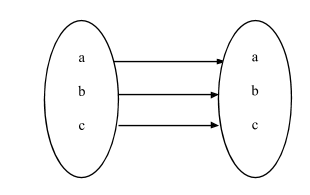QuestionAnswers

# A mapping is selected at random from all the mappings defined on the set. A consisting of three distinct elements. The probability that the mapping selected is one to one isA.$\dfrac{1}{9}$ B.$\dfrac{1}{3}$ C.$\dfrac{1}{4}$ D.$\dfrac{2}{9}$Hint:
If A and B are two non-empty sets, then a relation ‘f’ from set A to set B is said to be a function or mapping,
If every element of set A is associated with unique element of set B,
The mapping or function ‘f’ from A to B is denoted by f:A→A,
If f is a function from A to B and x ϵ A, then f(x) ϵ B where f(x) is called the image of x under f and x is called the preimage of f(x) under ‘f’.
Thus, for a mapping from A to B,
A and B should be non-empty.
Each element of A should have an image in B.
No element of ‘A’ should have more than one image in ‘B’.
Find the number of one-one maps and the total number of mappings from A to A.
$\therefore {\text{Required Probability = }}\dfrac{{{\text{Number of one - one map}}}}{{{\text{Total number of maps}}}}$

Complete step by step solution:
∴ A has 3-elements. Let it be a, b, c
∴ f :A→A∴Number of one to one mapping $= 3 \times 2 \times 1$
$= 6$
And total number of mapping $= 3 \times 3 \times 3$
$= 27$
∴ Probability that the mapping is one to one is $= \dfrac{{{\text{Number of one to one maps}}}}{{{\text{Total number of maps}}}}$
$= \dfrac{2}{9}$
∴ Option (D) is correct

Note:
Every mapping is a relation but every relation may not be a mapping. If a set has n-elements and a mapping f is defined on A. Therefore, number of one-to-one mapping is $\left| \!{\underline {\, n \,}} \right.$and the total number of mappings is${\left( n \right)^n}$. Some important points to be keep in mind:
Two or more elements of A may have the same image in B.
f: x →y means that under the function of ‘f’ from A to B, an element x of A has image y in B.
It is necessary that every f image is in B but there may be some elements in B which are not f images of any element of A.

Where There is a Will There is a Way EssayThe Difference Between an Animal that is A Regulator and One that is A ConformerLaughter is a Best Medicine EssayChanging the Period of a PendulumThe Making of a ScientistArea of a Sector of a Circle FormulaCBSE Class 11 Maths FormulasWhat is the Cell Envelope?What is the Placebo EffectNCERT Books Class 6 English a Pact with the SunImportant Questions for CBSE Class 6 English A Pact with The Sun Chapter 8 - A Pact with the SunImportant Questions for CBSE Class 11 Accountancy Chapter 11 - Accounts From Incomplete RecordsImportant Questions for CBSE Class 11 Business Studies Chapter 7 - Formation of a CompanyImportant Questions for CBSE Class 6 English A Pact with The Sun Chapter 1 - A Tale of Two BirdsImportant Questions for CBSE Class 6 English A Pact with The Sun Chapter 10 - A Strange Wrestling MatchImportant Questions for CBSE Class 11 Physics Chapter 4 - Motion in a PlaneImportant Questions for CBSE Class 6 English A Pact with The Sun Chapter 2 - The Friendly MongooseImportant Questions for CBSE Class 6 English A Pact with The SunImportant Questions for CBSE Class 6 English A Pact with The Sun Chapter 3 - The Shepherd's TreasureImportant Questions for CBSE Class 6 English A Pact with The Sun Chapter 6 - The Monkey and the CrocodileCBSE Class 10 Hindi A Question Paper 2020Hindi A Class 10 CBSE Question Paper 2009Hindi A Class 10 CBSE Question Paper 2015Hindi A Class 10 CBSE Question Paper 2016Hindi A Class 10 CBSE Question Paper 2012Hindi A Class 10 CBSE Question Paper 2010Hindi A Class 10 CBSE Question Paper 2007Hindi A Class 10 CBSE Question Paper 2013Hindi A Class 10 CBSE Question Paper 2008Hindi A Class 10 CBSE Question Paper 2014RD Sharma Class 12 Maths Solutions Chapter 32 - Mean and Variance of a Random VariableNCERT Solutions for Class 11 Maths ExercisesNCERT Solutions Class 11 English Woven Words Essay Chapter 5 What is a Good BookNCERT Solutions for Class 9 English Moments Chapter 8 - A House is Not a HomeRS Aggarwal Class 11 Solutions Chapter-16 Conditional Identities Involving the Angles of a TriangleRD Sharma Class 12 Solutions Chapter 32 - Mean and Variance of a Random Variable (Ex 32.2) Exercise 32.2RD Sharma Class 12 Solutions Chapter 32 - Mean and Variance of a Random Variable (Ex 32.1) Exercise 32.1NCERT Solutions for Class 11 Accountancy Chapter 11 Accounts from Incomplete RecordsRS Aggarwal Class 8 Mathematics Solutions for Chapter-18 Area of a Trapezium and a PolygonNCERT Solutions for Class 11 Business Studies - Chapter 7 - Formation of a Company